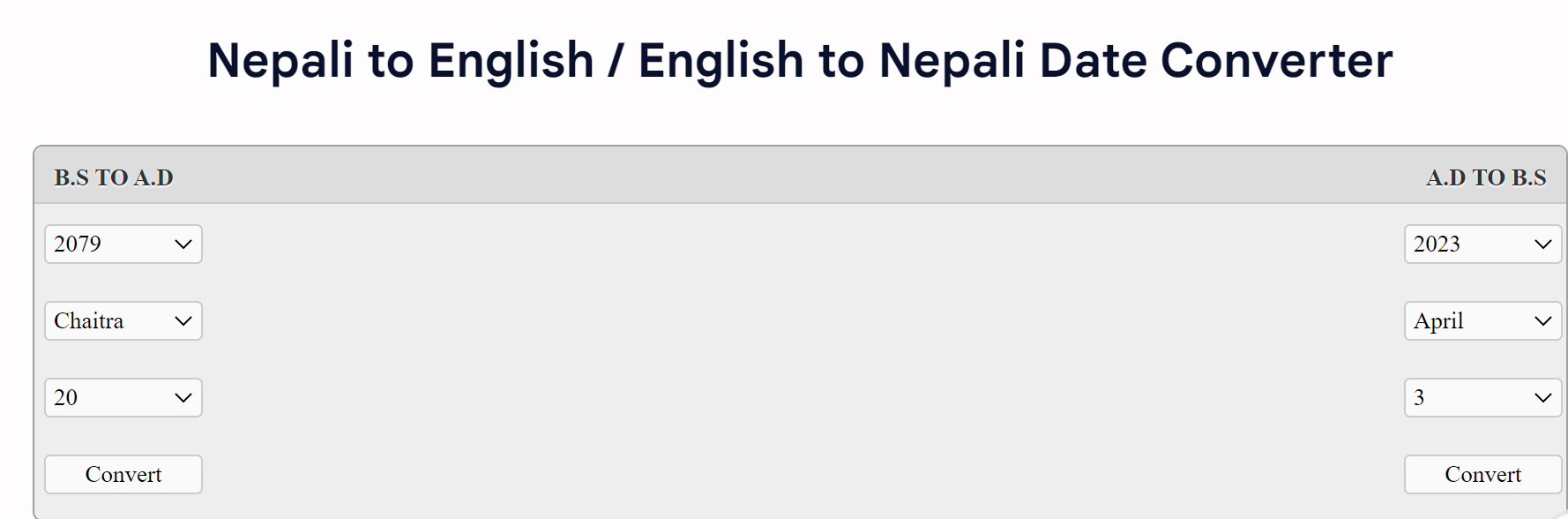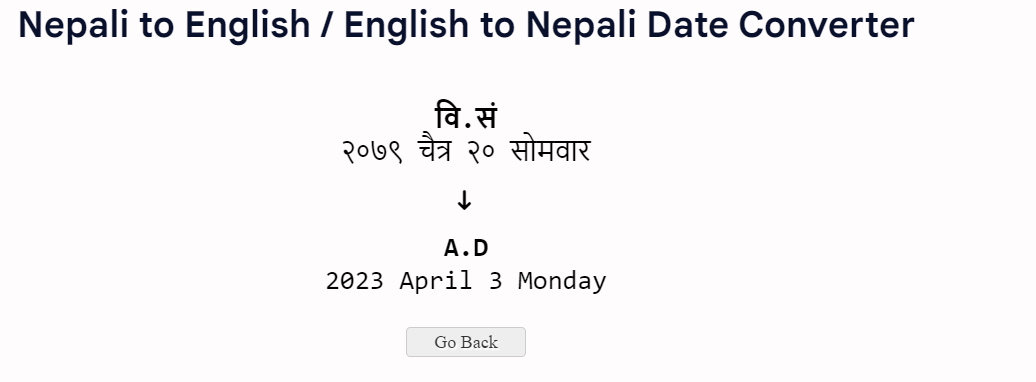# Nepali to English Date Converter (B.S. to A.D and A.D to B.S.)

Our Nepali Date Converter Helps to convert Nepali date (Bikram Sambat, B.S. ) date to English date (Gregorian Date, A.D. ) aka BS to AD and Vice Versa. This will help you to convert Nepali Date to English Date Easily.

## Nepali to English and English to Nepali Date Converter

Nepali English Date Converter Images:Result:### How to Convert Nepali Date to English Date?

To convert Nepali (BS) dates to English (AD), you can use our online date converter tool from above. Here are the steps to follow:

2. Select the Year, Month and Day
3. Press the "convert" button

Then, It will show the result of your date conversion.

### What is BS and AD?

BS or B.S. stands for Bikram Sambat, used primarily in the official Hindu Calendar in Nepal. Whereas AD or A.D. stands for Anno Domini, a label for numbering years after Christ's birth and widely used internationally.

### Formula to Convert BS to AD

The formula for converting a Bikram Sambat (BS) date to the Gregorian calendar (AD) is:

AD year = BS year - 57 years + (AD calendar year - BS calendar year) / 60

Let's take an example to understand this formula. Suppose we want to convert the Nepali date 2079-11-23 BS to the Gregorian calendar.

• The first step is to subtract 57 from the BS year. In this case, 2079 - 57 = 2022.
• Next, we need to calculate the difference between the BS calendar year and the AD calendar year. To do this, we subtract the AD calendar year (1) from the BS calendar year (11). This gives us 10 (11 -1 = 10).
• Since the Nepali calendar has a 12-year cycle and the Gregorian calendar has a 1-year cycle, we need to adjust for this difference by dividing the difference in years by 60. In this case, 10 / 60 = 0.1667.
• Finally, we add the result to the AD year (2022) to get the equivalent AD date. This gives us 2022 + 0.1667 = 2022.1667.
• Therefore, the equivalent AD date for the Nepali date 2079-11-23 BS is around April 7th, 2022.

### What are Covered in Nepali to English Date Converter tools?

In this post, Nepali to English Date Convertor tools We Covered as follows:

• Converter Nepali to English
• Date of Birth Converter
• Date Converter English to Nepali
• B.S. to A.D. Date Converter
• English to Nepali Date Converter
• Formula to Convert BS to AD

Site is Blocked
Sorry! This site is not available in your country.